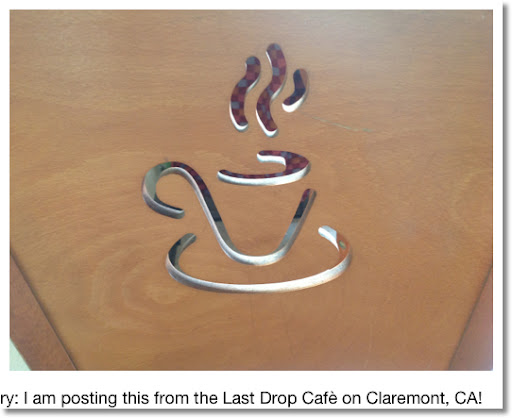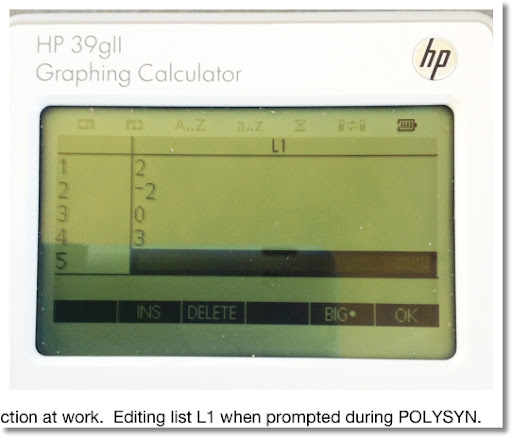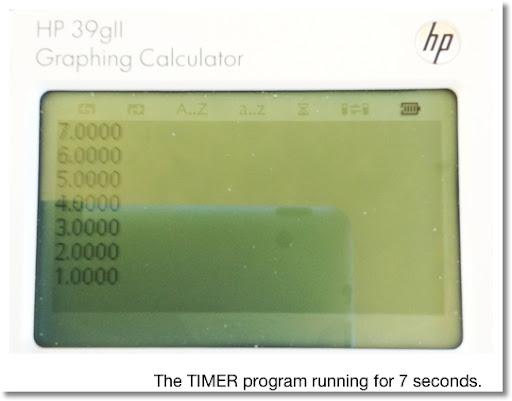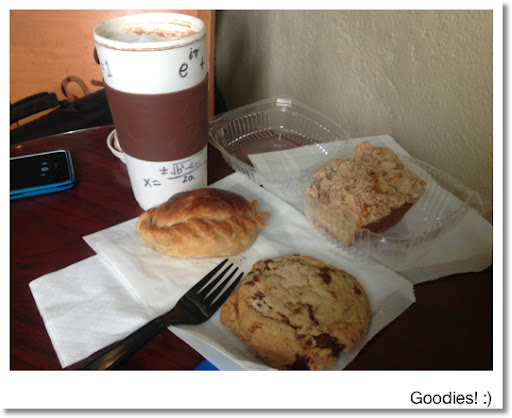## Saturday, March 16, 2013

### HP 39gii Programming Part 3: FOR LoopsThis blog entry: I am posting this from the Last Drop Cafè on Claremont, CA - in the college town near the Claremont Colleges. Quite a nice, cozy place. Good coffee too!

-------

Welcome to Part 3!

1. FOR Loops
2. Other Commands Used in this Lesson
3. The program CROOTS
4. The program POLYSYN
5. The program TIMER

1. FOR Loops

The FOR loop is good construct when you want to repeat a set of commands an amount of times. The commands may (or may not) involve a calculation involving a counter variable. For each pass, the value of counter is incremented (or decremented) until counter matches a target number.

An example is:

FOR K FROM 1 TO 4 DO
A:=A+K;
END;

The counter variable in this example is K. For the first pass, K=1. After 1 is added to A, then the loop ends. K increases by 1. For the second pass, K=2. On so on until the fourth pass where K=4. At that point, K is at its target value and the loop ends.

The increment can be any value you want. If you want the increment to be something other than 1, you can specify that by the STEP instruction. For example,

FOR K FROM 1 TO 4 STEP 0.5 DO
A:=A+K;
END;

Here we have specified the step size to be 0.5, which means that with each pass, K increases by 0.5 (from 1 to 1.5 to 2 to 2.5 and so on until K=4).

The FOR loop for the HP 39gii has four general formats.

Basic FOR loop: Increase counter by an increment of 1:

FOR counter FROM start_value TO end_value DO
insert commands here
END;

Keystrokes for this template (in the program editor): [ F6 ] (TMPLT) [ 4 ] [ 1 ]

FOR loop: Increase counter by an increment other than 1:

FOR counter FROM start_value TO end_value STEP increment DO
insert commands here
END;

Keystrokes for this template (in the program editor): [ F6 ] (TMPLT) [ 4 ] [ 2 ]

For loops with where the value of counter decreases, use the following two formats. Please note at the time of this posting, DOWNTO is an undocumented feature.

FOR loop: Decrease counter by a decrement of 1:

FOR counter FROM start_value DOWNTO end_value DO
insert commands here
END;

FOR loop: Decrease counter by a decrement other than 1:

FOR counter FROM start_value DOWNTO end_value STEP decrement DO
insert commands here
END;

I prefer just typing out the FOR-FROM-DOWNTO/TO-STEP-END structure manually.

2. Other Commands Used in this Lesson

The global variable HAngle. This is the master angle setting. Assigning the following values will result as:

1: Degrees Mode
2: Current Mode

How to find HAngle, if you don't want to type it: [Vars] [ F1 ] [ 4 ] [ 2 ]

CONCAT This command puts two lists together as one.

CONCAT(left list, right list)

Example: CONCAT({1,2,3},{5,6,8}) returns {1,2,3,5,6,8}.

Keystrokes: [Math] [ F1 ] [ 7 ] [ 1 ]

EDITLIST

Syntax: EDITLIST(L#);

This puts the calculator in list editing mode. The command edits the list L# where # is a digit 0-9. You can use a localized list name if you wish instead of the global variable L#.

WAIT

Syntax: WAIT(n);

If n=0, the calculator waits indefinitely. Press any key to have the calculator continue. This is great for freezing the screen to display certain results.

If n>0, the calculator waits n seconds before executing the next step. Useful in loops and displaying multiple bits of information. n does not have to be an integer.

Keystrokes: [SHIFT] [Math] [ F1 ] [ 5 ] [Vars]

The three programs CROOTS (Complex Roots), POLYSYN (Synthetic Division), and TIMER will demonstrate these commands.

3. The Program CROOTS

The first program to demonstrate the power of the FOR loop is the program CROOTS. This program finds all the complex roots of a given number. CROOTS finds all the Nth roots of Z and returns the answer in a list.

Input: CROOTS(Z,N)

The program CROOTS:

EXPORT CROOTS(Z,N)
BEGIN
LOCAL L3,K;
HAngle:=0;
L3:={};
FOR K FROM 0 TO N-1 DO
L3:=CONCAT(L3, {N NTHROOT (ABS(Z)) * e^( i * ( ARG(Z) + 2 * K * π )/N)});
END;
RETURN L3;
END;

Examples (answers are rounded to 5 decimal places)

CROOTS(4,2) returns [2, -2 - 2.5335E-12 * i]. In essence, [2, -2]

CROOTS(-1, 3) returns [0.5 + 0.86603*i, -1*, 0.5 - 0.86603*i].

* -1 - 1.26676E-12*i

4. The Program POLYSYN

Description of POLYSYN:

POLYSYN is a program that divides the polynomial

p(x) / (x-R)

by the use of synthetic division. POLYSYN has no pass-through arguments. The program prompts L1, which is a list of coefficients of descending power to the constants. Please include zeroes whenever necessary. The value of R is also prompted.

The result is a list of coefficients, with the last term the remainder

EXPORT POLYSYN()
BEGIN
LOCAL K,S,T;
EDITLIST(L1);
INPUT(R,"P(X)/(X-R)");
L2:=L1;
S:=SIZE(L1);
T:=S-1;
FOR K FROM 1 TO T DO
L2(K+1):=R * L2(K) + L1(K+1);
END;
MSGBOX("LAST TERM=REMAINDER");
RETURN L2;
END;

L1, R, and L2 are permanently updated.

Example 1: (21x^2 + 42x + 144)/(x - 12) = 21x + 294 + 3672/(x-12)

Inputs:
L1 = {21, 42, 144}
R = 12

Output:
L2 = {21, 294, 3672}

Example 2: (x^3 - 5x + 1)/(x + 5) = x^2 - 5x + 20 - 99/(x + 5)

Input:
L1 = {1, 0, -5, 1}
R = -5

Output:
L2 = {1, -5, 20, -99}5. The program TIMER

TIMER demonstrates a FOR loop with DOWNTO. The program takes one pass-through argument: the number of seconds.

The program TIMER:

EXPORT TIMER(N)
BEGIN
LOCAL K;
FOR K FROM N DOWNTO 1 DO
PRINT(K);
WAIT(1);
END;
END;In Part 4 of the HP 39gii Series, we will deal with REPEAT And UNTIL.

As always, thank you - I am grateful for your comments and following this blog. Have a great day!

Eddie

This blog is property of Edward Shore. 2013